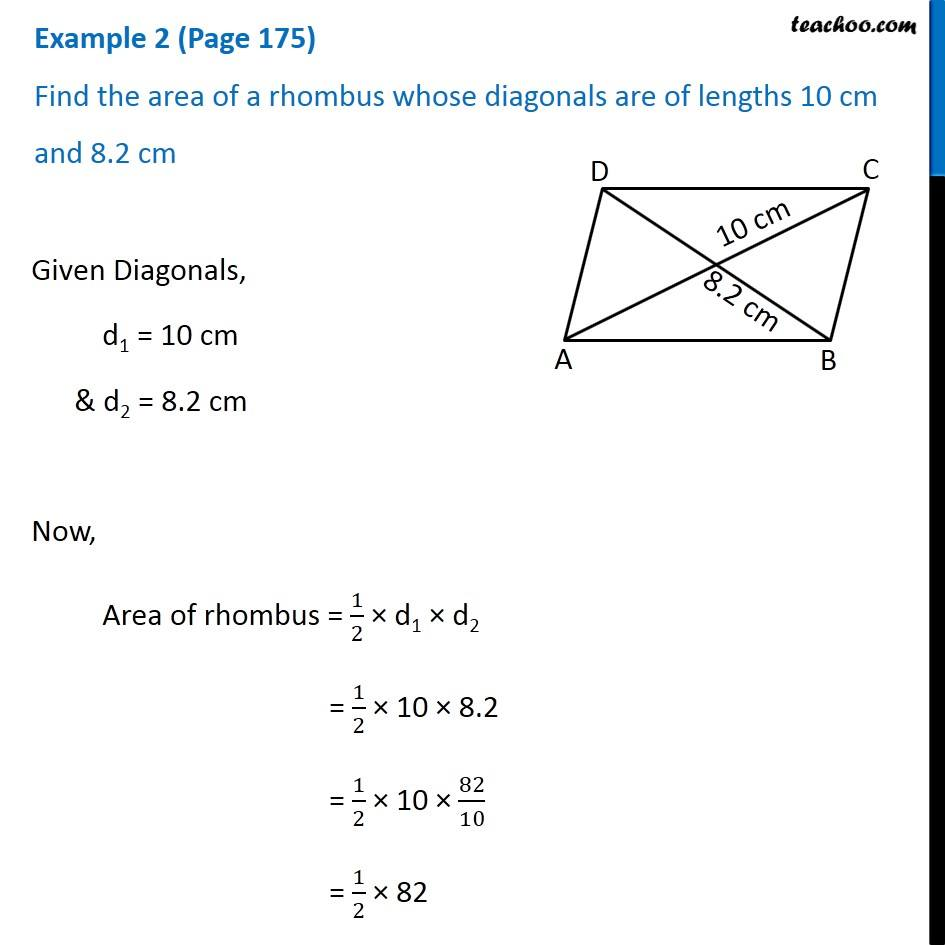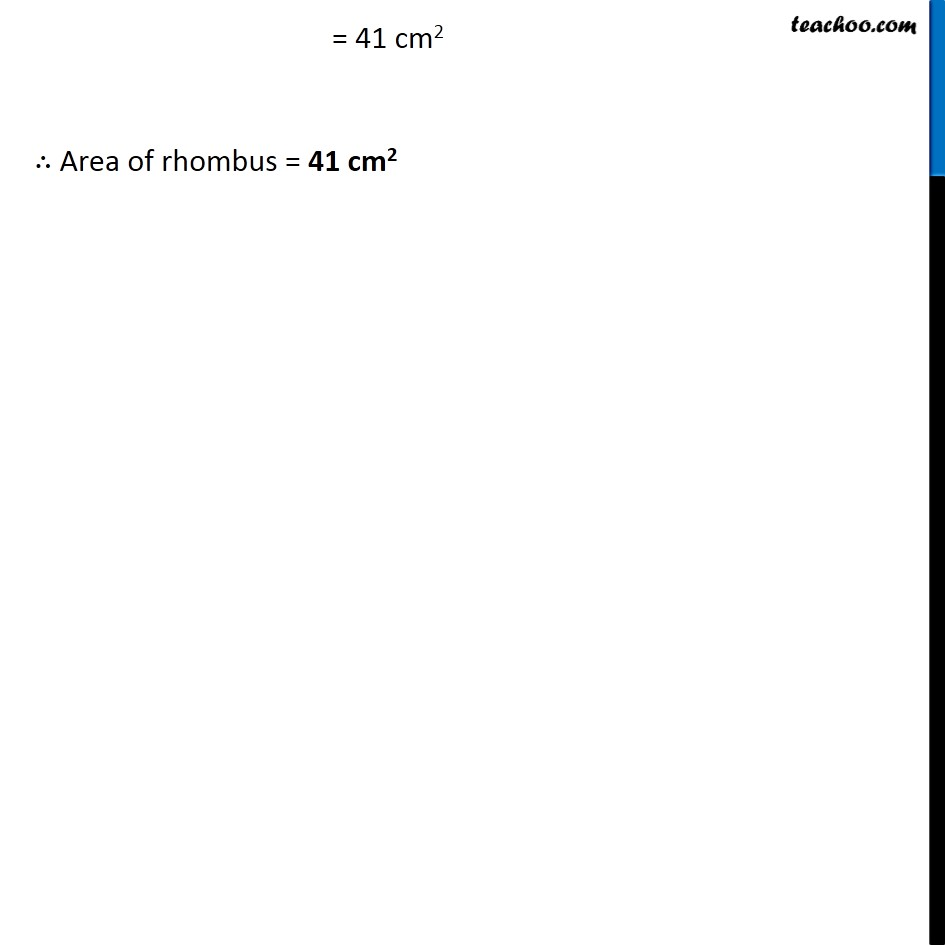Subscribe to our Youtube Channel - https://you.tube/teachoo

1. Chapter 11 Class 8 Mensuration
2. Concept wise
3. Area of Rhombus

Transcript

Example 2 (Page 175) Find the area of a rhombus whose diagonals are of lengths 10 cm and 8.2 cm Given Diagonals, d1 = 10 cm & d2 = 8.2 cm Now, Area of rhombus = 1/2 × d1 × d2 = 1/2 × 10 × 8.2 = 1/2 × 10 × 82/10 = 1/2 × 82 = 41 cm2 ∴ Area of rhombus = 41 cm2

Area of Rhombus